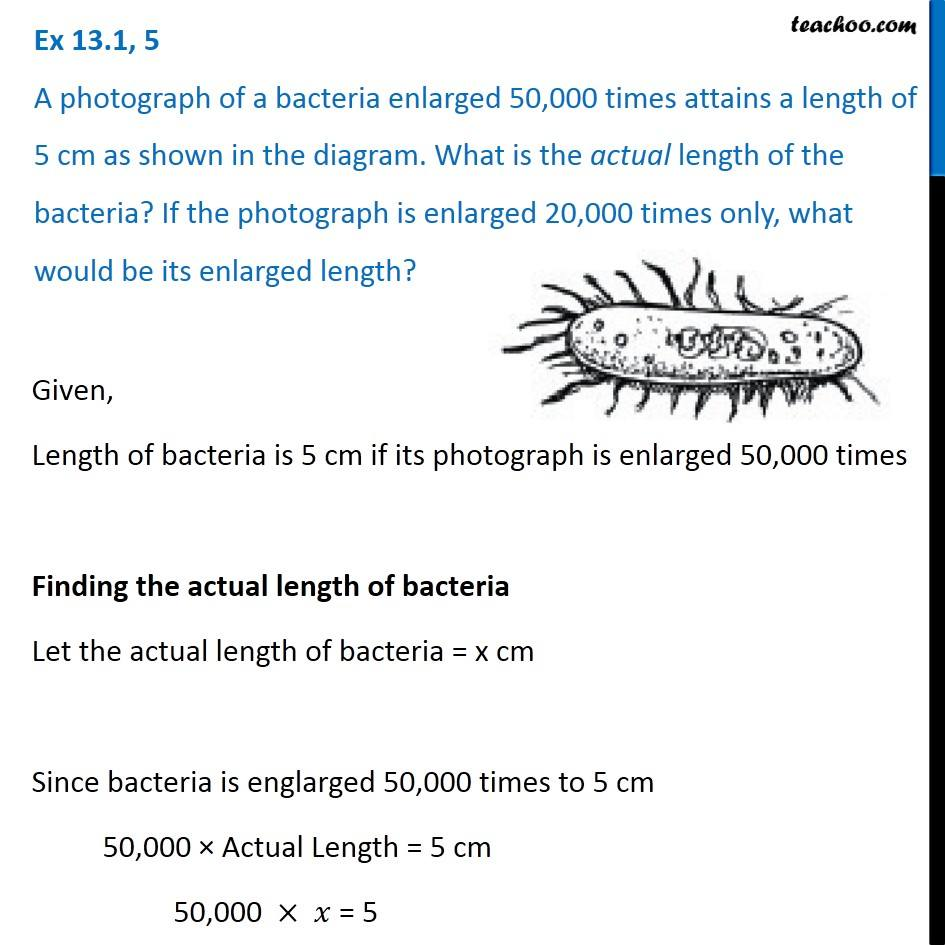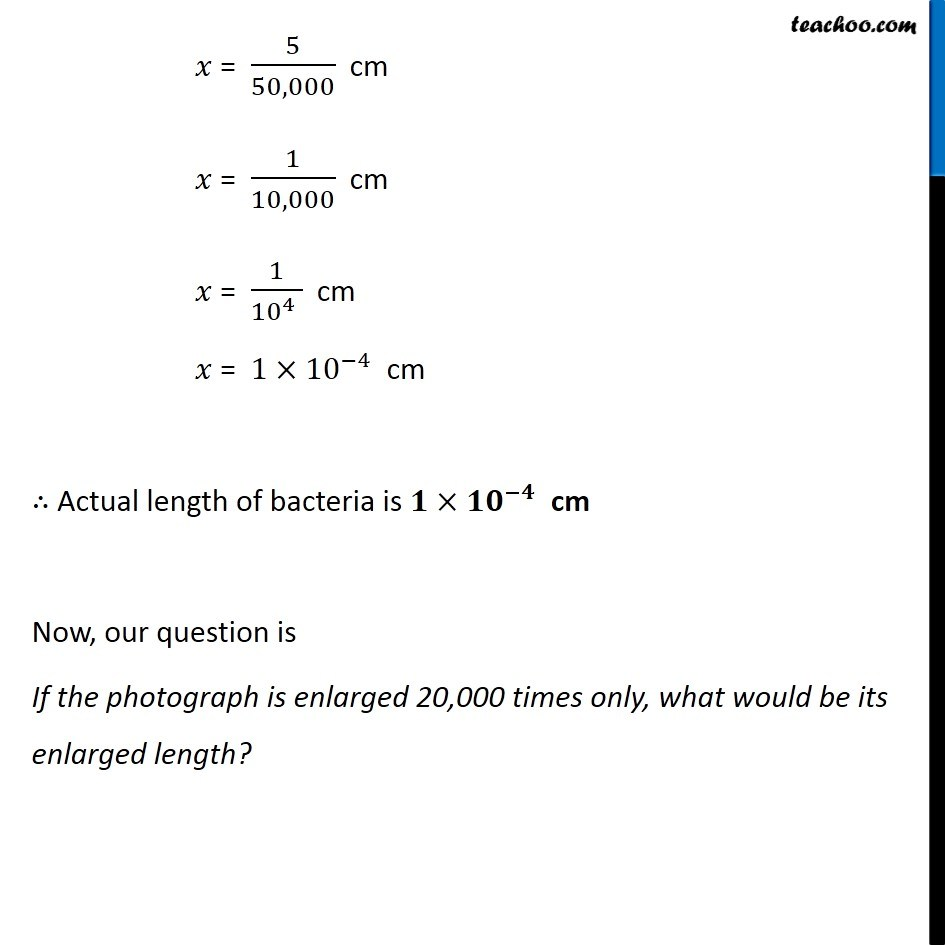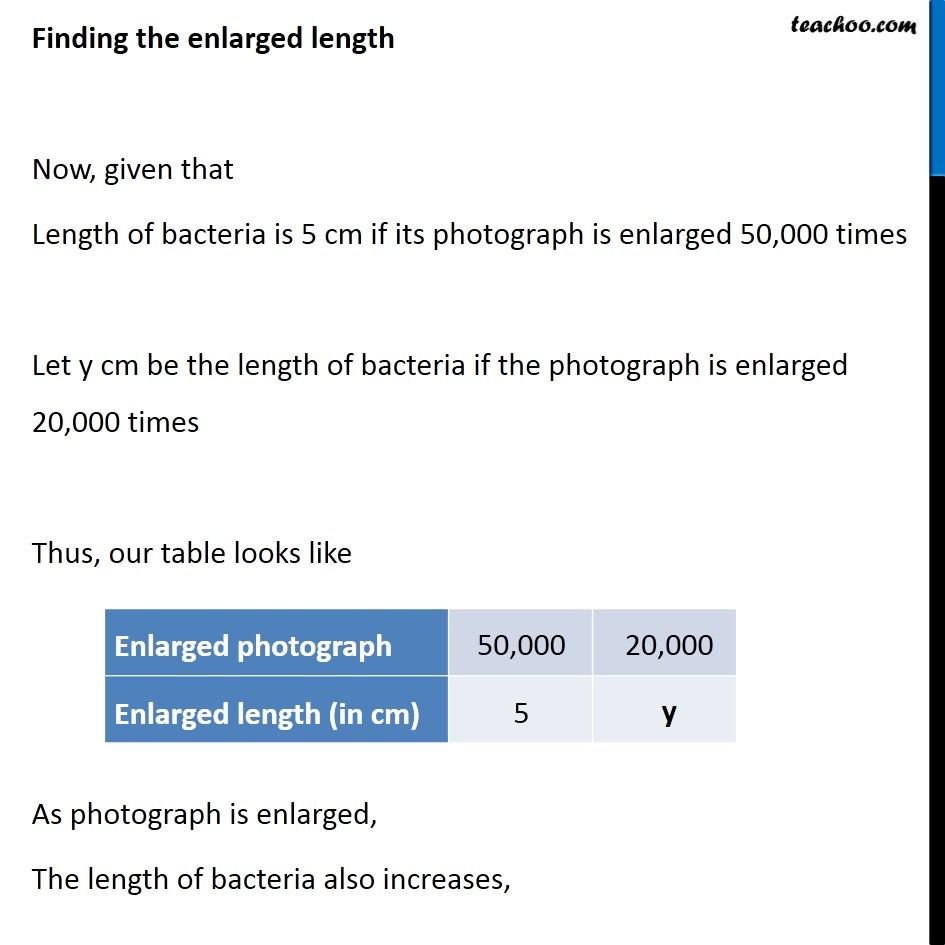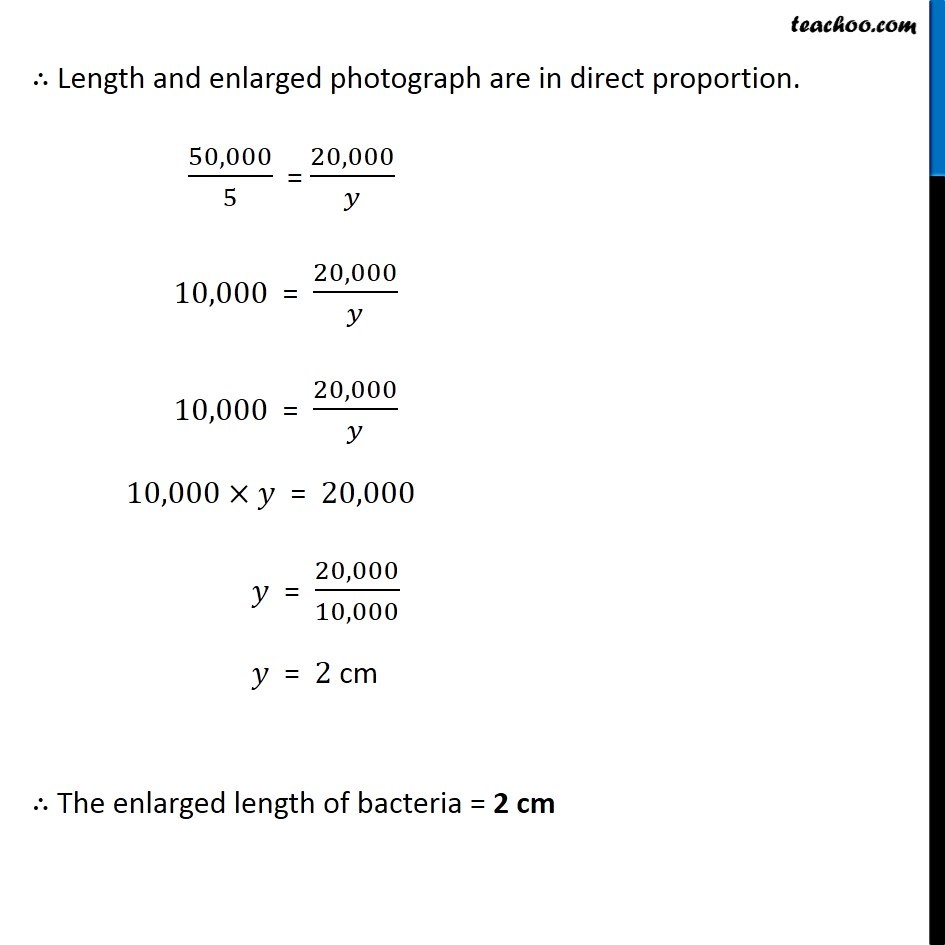Ex 13.1

Chapter 13 Class 8 Direct and Inverse Proportions
Serial order wiseGet live Maths 1-on-1 Classs - Class 6 to 12

### Transcript

Ex 13.1, 5 A photograph of a bacteria enlarged 50,000 times attains a length of 5 cm as shown in the diagram. What is the actual length of the bacteria? If the photograph is enlarged 20,000 times only, what would be its enlarged length? Given, Length of bacteria is 5 cm if its photograph is enlarged 50,000 times Finding the actual length of bacteria Let the actual length of bacteria = x cm Since bacteria is englarged 50,000 times to 5 cm 50,000 × Actual Length = 5 cm 50,000 × 𝑥 = 5 𝑥 = 5/50,000 cm 𝑥 = 1/10,000 cm 𝑥 = 1/(〖10〗^4 ) cm 𝑥 = 1×〖10〗^(−4) cm ∴ Actual length of bacteria is 𝟏×〖𝟏𝟎〗^(−𝟒) cm Now, our question is If the photograph is enlarged 20,000 times only, what would be its enlarged length? Finding the enlarged length Now, given that Length of bacteria is 5 cm if its photograph is enlarged 50,000 times Let y cm be the length of bacteria if the photograph is enlarged 20,000 times Thus, our table looks like As photograph is enlarged, The length of bacteria also increases, ∴ Length and enlarged photograph are in direct proportion. 50,000/5 = 20,000/𝑦 10,000 = 20,000/𝑦 10,000 = 20,000/𝑦 10,000×𝑦 = 20,000 𝑦 = 20,000/10,000 𝑦 = 2 cm ∴ The enlarged length of bacteria = 2 cm